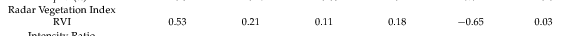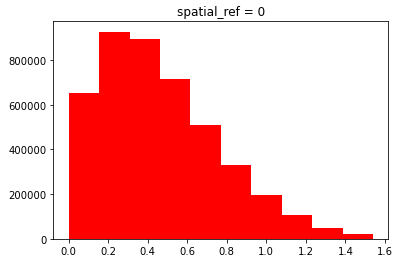# Sentinel-1 Radar Vegetation Index in python

Hi everyone,

I have created the Sentinel-1 GRD backscatter ratio based on the backscatter coefficients VV and VH gamma nought. Currently, I am planning to calculate the Radar Vegetation Index (RVI) based on the VV and VH polarisations. But I am facing the problems in calculations via different ways in python.

1. In my first way, I just took the backscatter coefficients from the preprocessed Sentinel-1 GRD image and computed based on the formula suggested in this paper.

I used the following format

`> ((4*abs(backscatter_pol))/(abs(backscatter_pol)+abs(backscatter_pol)))`

where backscatter_pol is the preprocessed image with gamma_VV and gamma_VH as bands which are in dB unit.
This is the obtained image.

The value ranges from 0 to 4.

1. In my second way, I followed this article
`rvi1 = (np.sqrt(((abs(backscatter_pol))/(abs(backscatter_pol)+abs(backscatter_pol)))))*((4*abs(backscatter_pol))/(abs(backscatter_pol)+abs(backscatter_pol)))`
obtained result is

Here the value ranges from 0 to ~1.5 .

So, I have five questions

1. Whether the values are correct are not for the Radar Vegetation Index based on the above suggested paper and article?
2. Is it necessary that not to convert from linear to dB while preprocessing for RVI calculation?
3. Is this the same case when using in SLC?
4. What is the general range of the RVI? please let me know any literatures about the ranges.
5. Is there any workflow in pyroSAR regarding the RVI? Because I haven’t seen the parameters of BandMaths operator in snap command-line. If there is any suggestion regarding the use of .xml file please let me know how to include it in my pyroSAR script.

A quick google search brought me this sales document, https://servirglobal.net/Portals/0/Documents/Articles/2019_SAR_Handbook/SAR_Vegetation%20Indices_one-pager.pdf that suggests the values should be 0 to 1 for the RVI

Page 13 of your first article suggests that RVI should be between 0 and 1 also, just by looking at their values,Your second reference says, “The index changes from almost 0 to close to 1 as crop advanced.”

So I think there is an error in your process and your values are incorrect in both cases. I’m not sure the answers to your other questions.

Dear @cndnflyr ,

Thank you for your reply. So the answer will become correct without changing the values from linear to dB while preprocessing the sentinel-1 GRD, is it?

Today, I computed the linear backscatter coefficient (gamma nought) of Sentinel-1 GRD (didn’t converted to dB scale) image on 10th February 2021 and applied the RVI formula based on this article and I got the following output.

where the value is again between 0 and ~1.5 and the histogram is as follows which is a huge difference from the previous result.So, is this right?

Greetings,
Karlmarx